# Project Euler

What is Project Euler?
Project Euler is a series of challenging problems that require mathematical and programming skills. Somebody who enjoys learning new area of mathematics, project Euler is going to be a fun journey.

Where are the problems ?
The problems are right here in their official archive.

Let’s solve a problem from the archive and understand its complexity. Randomly I have chosen Problem no 116.
Problem 116 : Red, green or blue tiles
Problem Statement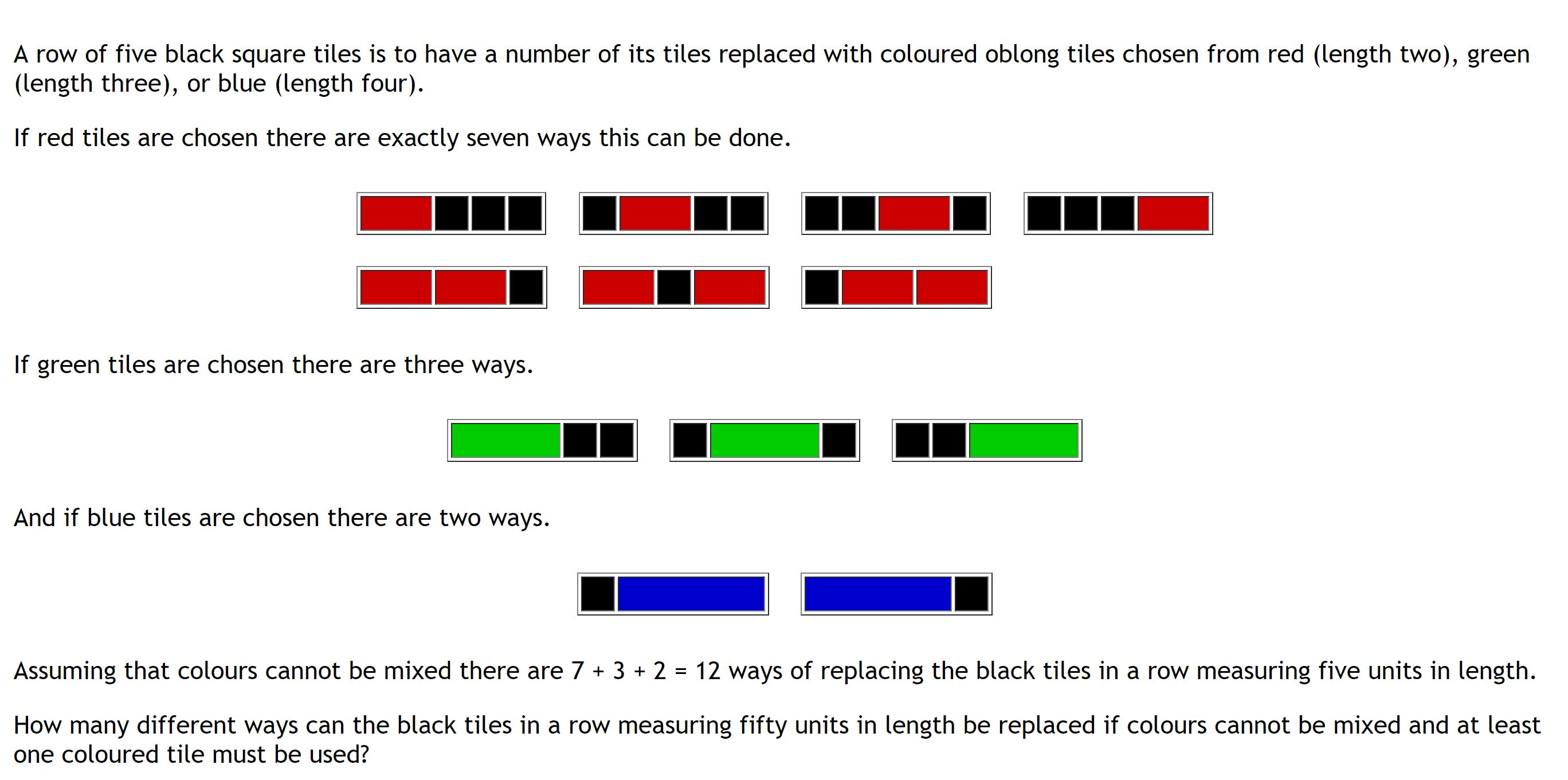Solution: [ IT IS ADVISED TO TRY YOURSELF FIRST]
A red tile is of length 2, green is of length 3 and blue is of length 4.

Since, we need to count total ways for 50 units of black colored square tiles, say k = 50.

```def E_116(i, k):
ways =  * i +  * (k-i+1)
for j in range(i, k+1):
ways[j] += ways[j - 1] + ways[j - i]
return ways[k] - 1
```

Here, we are initializing our function E_116() which holds the logic of the solution to the problem.The function E_116() has two parameters i = number of black coloured square tiles covered by the new coloured (red, green or blue) tiles and k = total number of black coloured square tiles.
In the function,

`ways =  * i +  * (k-i+1)`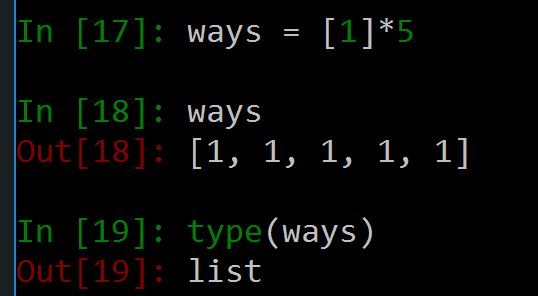So, ways is a list which holds the total number of ways which the i length block can cover 50 black coloured square tiles.For e.g: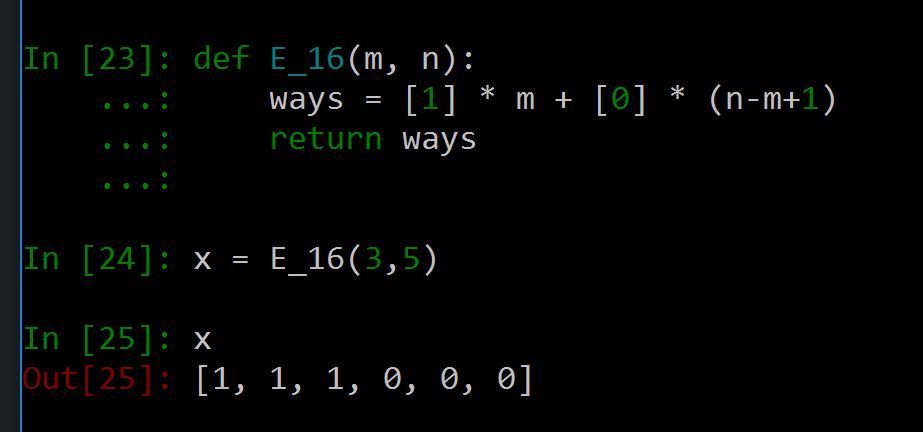In the above example x = [1, 1, 1, 0, 0, 0] , has 1 (x3) and 0 (x3) , where 1 represents possible solution case and 0 represents failure.As we can compare for i = 3 and k = 5 from the question, we get total 3 possible ways.Hence there are 1 (x3) in the list, ways.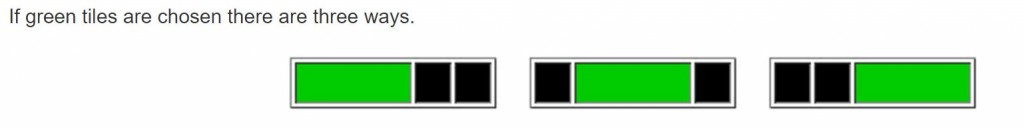```for j in range(i, k+1):
ways[j] += ways[j - 1] + ways[j - i]
```

Using the for loop we are iterating from i to 50, we have written k+1 since iterating in the loop through range() will exclude the last case.In ways[j] += ways[j – 1] + ways[j – i], we are updating the jth index of the list ways with the summation of (j-1) and (j+i)th index’s value and also the jth index value (Since +=).
These are the possible values of j, for i=3 and k =5.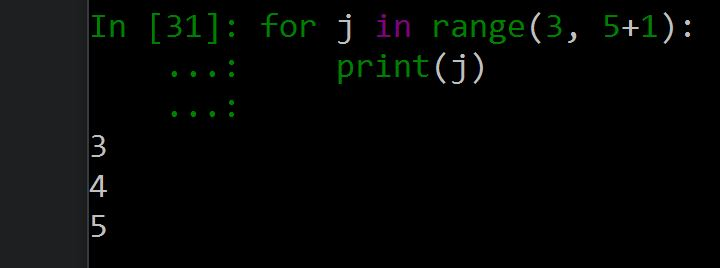Finally we return our list as return ways[k] – 1.So, for i=3 and k=5, this gives the solution(i.e, Ans = 3)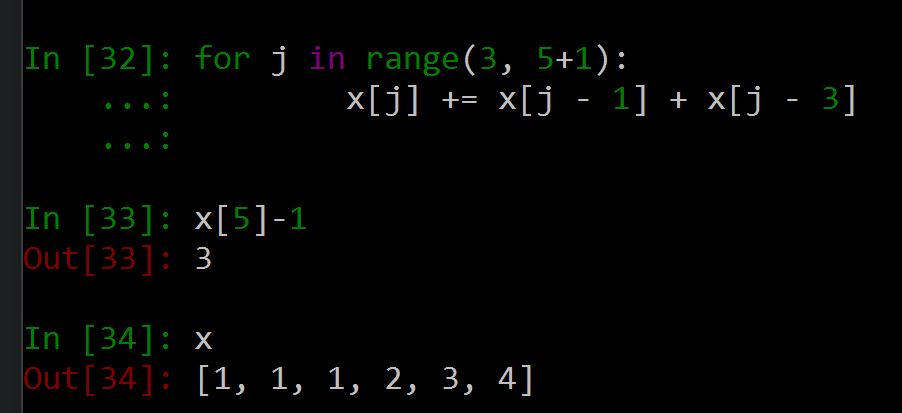Lastly in our code,

```print("Number of black tiles =", k, "units")
print("Number of ways to fill:", E_116(2, k) + E_116(3, k) + E_116(4, k))
```

We print away our result using the print() function. The first statement prints Number of black tiles = 50 and the second statement prints Number of ways to fill: 20492570929 which is the desired answer to the problem.

 `# Project Euler Problem 116 ` ` `  `k ``=` `50` `def` `E_116(i, k): ` `    ``ways ``=` `[``1``] ``*` `i ``+` `[``0``] ``*` `(k``-``i``+``1``) ` `    ``for` `j ``in` `range``(i, k``+``1``): ` `        ``ways[j] ``+``=` `ways[j ``-` `1``] ``+` `ways[j ``-` `i] ` `    ``return` `ways[k] ``-` `1` ` `  `print``(``"Number of black tiles ="``, k, ``"units"``) ` `print``(``"Number of ways to fill:"``, E_116(``2``, k) ``+` `E_116(``3``, k) ``+` `E_116(``4``, k)) `

Resources:

This article is contributed by Amartya Ranjan Saikia. If you like GeeksforGeeks and would like to contribute, you can also write an article using contribute.geeksforgeeks.org or mail your article to contribute@geeksforgeeks.org. See your article appearing on the GeeksforGeeks main page and help other Geeks.